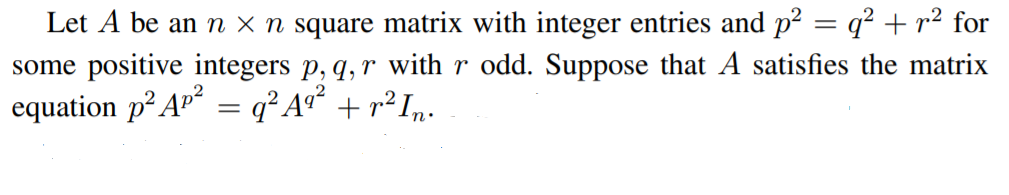# Revision history [back]

### How to construct a class of graphs satisfying a given matrix equation.Here we know that $A=I_n$ satisfies the given matrix equation. But can we find other non trivial matrix ($\neq I_n$). In other words, can we construct a class of graphs satisfying the given matrix equation.

### How to construct a class of graphs satisfying a given matrix equation.Here we know that $A=I_n$ satisfies the given matrix equation. But can we find other non trivial matrix ($\neq I_n$). In other words, can we construct a class of graphs satisfying the given matrix equation.Here we know that $A=I_n$ satisfies the given matrix equation. But can we find other non trivial matrix ($\neq I_n$). In other words, can we construct a class of graphs matrices satisfying the given matrix equation.